JMSLTM Numerical Library 7.2.0
com.imsl.stat

## Class ClusterKNN

• All Implemented Interfaces:
Serializable, Cloneable

```public class ClusterKNN
extends Object
implements Serializable, Cloneable```

Perform a k-Nearest Neighbor classification.

`ClusterKNN` implements an algorithm to classify objects based on a training set. Among the simpler algorithms for classification, classifying a new object is essentially a majority vote of its closest `k` neighbors. `k` must be a positive integer and is typically small and odd. The method is straightforward in that the distance from the new point to every point in the training set is computed and sorted. The `k` closest points are examined and the new object is assigned to the class that is most common in that set. For the case `k = 1` the object is assigned to the class of its nearest neighbor.

The default distance method is the Euclidean distance, but other options are available by using the `setDistanceMethod` method. The supported methods are:

 `method` Description `L2_NORM` The Euclidean distance method,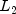norm, defined as the sum of the squares of the difference of each coordinate. (Default) `L1_NORM` The rectilinear norm or city block method,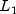norm, defined as the sum of the absolute values of the difference of each coordinate. This is most useful for integer input data. `INFINITY_NORM` The Chebyshev distance method,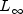norm, defined as the maximum of the absolute values of the difference of each coordinate.

For cases where the data are poorly scaled, it may be necessary to normalize the input data first. For example, if in a 2D space the X values range from 0 to 1 and the Y values, from 0 to 1000, the distance calculations will be dominated by the Y coordinate unless they are normalized.

ClusterKNN Example 1, Serialized Form
• ### Field Summary

Fields
Modifier and Type Field and Description
`static int` `INFINITY_NORM`
Indicates the distance is computed using thenorm method.
`static int` `L1_NORM`
Indicates the distance is computed using thenorm method.
`static int` `L2_NORM`
Indicates the distance is computed using thenorm, or Euclidean distance measurement.
• ### Constructor Summary

Constructors
Constructor and Description
```ClusterKNN(double[][] x, int[] c)```
Constructor for `ClusterKNN`.
• ### Method Summary

Methods
Modifier and Type Method and Description
`int[]` ```classify(double[][] value, int k)```
Classify a set of observations using `k` nearest neighbors.
`int` ```classify(double[] value, int k)```
Classify an observation using `k` nearest neighbors.
`void` `setDistanceMethod(int method)`
Sets the distance calculation method to be used.
• ### Methods inherited from class java.lang.Object

`clone, equals, finalize, getClass, hashCode, notify, notifyAll, toString, wait, wait, wait`
• ### Field Detail

• #### INFINITY_NORM

`public static final int INFINITY_NORM`
Indicates the distance is computed using thenorm method. This is also known as the maximum difference or Chebyshev distance.
Constant Field Values
• #### L1_NORM

`public static final int L1_NORM`
Indicates the distance is computed using thenorm method. Also known as rectilinear distance or city block distance, it is most useful for integer input data.
Constant Field Values
• #### L2_NORM

`public static final int L2_NORM`
Indicates the distance is computed using thenorm, or Euclidean distance measurement.
Constant Field Values
• ### Constructor Detail

• #### ClusterKNN

```public ClusterKNN(double[][] x,
int[] c)```
Constructor for `ClusterKNN`.
Parameters:
`x` - A `double` matrix containing the known `x.length` observations of ```x.length ``` variables.
`c` - An `int` array containing the categories for the `x.length` observations. All integer values are valid.
• ### Method Detail

• #### classify

```public int[] classify(double[][] value,
int k)```
Classify a set of observations using `k` nearest neighbors.
Parameters:
`value` - A `double` matrix of `value.length` observations and `x.length` variables to classify.
`k` - An `int` containing the number of nearest neigbors to use. An odd value is recommended.
Returns:
An `int` array containing the cluster to which each of the observations belong.
• #### classify

```public int classify(double[] value,
int k)```
Classify an observation using `k` nearest neighbors.
Parameters:
`value` - A `double` array of `x.length` variables containing the observations to classify.
`k` - An `int` containing the number of nearest neigbors to use. An odd value is recommended.
Returns:
An `int` containing the cluster to which the observation belongs.
• #### setDistanceMethod

`public void setDistanceMethod(int method)`
Sets the distance calculation method to be used.
Parameters:
`method` - An `int` identifying the distance calculation method to be used. By default, `method` = `L2_NORM`.

 method Description `L2_NORM`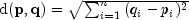`L1_NORM`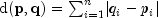`INFINITY_NORM`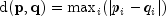JMSLTM Numerical Library 7.2.0